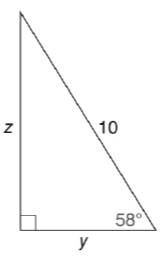Chapter 11.3, Problem 17EElementary Geometry For College St...

7th Edition
Alexander + 2 others
ISBN: 9781337614085

Solutions

Chapter
SectionElementary Geometry For College St...

7th Edition
Alexander + 2 others
ISBN: 9781337614085
Textbook Problem

In Exercises 15 to 20, use the sine, cosine, or tangent ratio to find the lengths of the indicated sides to the nearest tenth of a unit.To determine

To find:

The lengths of the indicated sides to the nearest tenth of a unit using the sine, cosine or tangent ratio.

Explanation

Given:

The triangle with the indicated sides is shown below.

Definition used:

The trigonometric ratios:

Calculation:

Let α=58.

From the figure we see that opposite=z,adjacent=y,hypotenuse=10.

We need to find the values of z, y.

We have sinα=oppositehypotenuse

sin58=z10

cos58=y10

Let us use Ti-83 calculator to find the value of sin58,cos58.

Press ON in Ti-83 calculator.

Since we need to find the value of degree, we need to change the mode to degrees.

Press MODE button and use arrow keys to select the “Degree” mode.

Let us find the values, correct to four decimal places.

Since we need the values of sin58,cos58 correct to four decimal places, in MODE, using arrow keys choose the value of “Float” as 4

Still sussing out bartleby?

Check out a sample textbook solution.

See a sample solution

The Solution to Your Study Problems

Bartleby provides explanations to thousands of textbook problems written by our experts, many with advanced degrees!

Get Started

Solving Equations Using nth Roots Solve the equation. 90. z6 1 = 0

Precalculus: Mathematics for Calculus (Standalone Book)

Find the following products (2+4i)(3i)

Trigonometry (MindTap Course List)

Using for |x| < 1 and differentiation, find a power series for .

Study Guide for Stewart's Single Variable Calculus: Early Transcendentals, 8th

True or False: div curl F = 0.

Study Guide for Stewart's Multivariable Calculus, 8th

In Exercises 1-18, construct a truth table for each compound proposition. (pq)(~p)

Finite Mathematics for the Managerial, Life, and Social Sciences